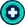This is a documentation on how the Pixies Infobox can be used.

Usage

{{SuitStatsUS
| name =
| class =
| CRT% =
| CRIT =
| CNTR =
| STNΩ =
| FRZΩ =
| SILΩ =
| ACDΩ =
<!-- Rank US -->
| iconname-US =
| height-US =
| weight-US =
| output-US =
| HP1 =
| MP1 =
| ATK1 =
| DEF1 =
| ACC1 =
| EVA1 =
| HP30 =
| MP30 =
| ATK30 =
| DEF30 =
| ACC30 =
| EVA30 =
<!-- Rank US+1 -->
| iconname-US1 =
| height-US1 =
| weight-US1 =
| output-US1 =
| HP31 =
| MP31 =
| ATK31 =
| DEF31 =
| ACC31 =
| EVA31 =
| HP40 =
| MP40 =
| ATK40 =
| DEF40 =
| ACC40 =
| EVA40 =
<!-- Rank US+2 -->
| iconname-US2 =
| height-US2 =
| weight-US2 =
| output-US2 =
| HP41 =
| MP41 =
| ATK41 =
| DEF41 =
| ACC41 =
| EVA41 =
| HP50 =
| MP50 =
| ATK50 =
| DEF50 =
| ACC50 =
| EVA50 =
<!-- Rank US+3 -->
| iconname-US3 =
| height-US3 =
| weight-US3 =
| output-US3 =
| HP51 =
| MP51 =
| ATK51 =
| DEF51 =
| ACC51 =
| EVA51 =
| HP60 =
| MP60 =
| ATK60 =
| DEF60 =
| ACC60 =
| EVA60 =
}}

it is important to ensure all fields are filled.

• name: Name of the Suit.
• class: Class of the suit. Must be Assault, Support, Sniper, or Bombardier
• TBA

Example

Momentia (US)Costlv.1 lv.30 CRT% 11% 52
Hp 1 30 Crit 12% Height
Mp 1 30 CNTR 13% 11mm
ATK 1 30 STNΩ 14% Weight
DEF 1 30 FRZΩ 15% 123tt
ACC 1 30 SILΩ 16% Output
EVA 1 30 ACDΩ 17% 4420kwkw
Momentia (US+1)Costlv.31 lv.40 CRT% 11% 52
Hp 31 40 Crit 12% Height
Mp 31 40 CNTR 13% 11mm
ATK 31 40 STNΩ 14% Weight
DEF 31 40 FRZΩ 15% 123tt
ACC 31 40 SILΩ 16% Output
EVA 31 40 ACDΩ 17% 4420kwkw
Momentia (US+2)Costlv.41 lv.50 CRT% 11% 52
Hp 41 50 Crit 12% Height
Mp 41 50 CNTR 13% 10m
ATK 41 50 STNΩ 14% Weight
DEF 41 50 FRZΩ 15% 12t
ACC 41 50 SILΩ 16% Output
EVA 41 50 ACDΩ 17% 2450kw

{{SuitStatsUS
| name = Momentia
| class = Support
| CRT% = 11
| CRIT = 12
| CNTR = 13
| STNΩ = 14
| FRZΩ = 15
| SILΩ = 16
| ACDΩ = 17
<!-- Rank US -->
| iconname-us = MomentiaUSicon
| height-US =  11m
| weight-US = 123t
| output-US = 4420kw
| HP1 = 1
| MP1 = 1
| ATK1 = 1
| DEF1 = 1
| ACC1 = 1
| EVA1 = 1
| HP30 = 30
| MP30 = 30
| ATK30 = 30
| DEF30 = 30
| ACC30 = 30
| EVA30 = 30
<!-- Rank US+1 -->
| iconname-US1 = MomentiaUS1icon
| height-US1 =  11m
| weight-US1 = 123t
| output-US1 = 4420kw
| HP31 = 31
| MP31 =  31
| ATK31 = 31
| DEF31 = 31
| ACC31 = 31
| EVA31 = 31
| HP40 = 40
| MP40 = 40
| ATK40 = 40
| DEF40 = 40
| ACC40 = 40
| EVA40 = 40
<!-- Rank US+2 -->
| iconname-US2 = MomentiaUS2icon
| height-US2 =  10m
| weight-US2 = 12t
| output-US2 = 2450kw
| HP41 = 41
| MP41 = 41
| ATK41 = 41
| DEF41 = 41
| ACC41 = 41
| EVA41 = 41
| HP50 = 50
| MP50 = 50
| ATK50 = 50
| DEF50 = 50
| ACC50 = 50
| EVA50 = 50
<!-- Rank US+3 -->
| iconname-US3 = MomentiaUS3icon
| height-US3 =  10m
| weight-US3 = 12t
| output-US3 = 2450kw
| HP51 = 51
| MP51 =  51
| ATK51 = 51
| DEF51 = 51
| ACC51 = 51
| EVA51 = 51
| HP60 = 60
| MP60 =  60
| ATK60 = 60
| DEF60 = 60
| ACC60 = 60
| EVA60 = 60
}}